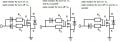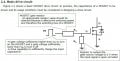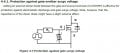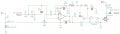# Driving a mosfet with Comparator

#### ericgibbs

Joined Jan 29, 2010
17,419
hi anish,
Look at these changes to your circuit.
Note you had V1 with zero output impedance so how could the hystersis work.?
E

Update:
Make V1 = 1.85v

#### Attachments

• 2 KB Views: 14
• 48.9 KB Views: 46

#### Papabravo

Joined Feb 24, 2006
20,132
You're going to be greatly surprised when you try to drive a MOSFET with the wimpy output of the comparator. Hint: it can sink more current than it can source. This means painfully slow turn on and potentially fatal overheating.

#### ArakelTheDragon

Joined Nov 18, 2016
1,355
"50mA" is something standard for a comparator maximum.

#### anishkgt

Joined Mar 21, 2017
549
You're going to be greatly surprised when you try to drive a MOSFET with the wimpy output of the comparator. Hint: it can sink more current than it can source. This means painfully slow turn on and potentially fatal overheating.
So would it be a mosfet driver before the mosfet ? or are there comparators that can drive mosfets at 5v? I have three comparators comparing voltage from a supercap and truning a mosfet on when voltage goes below a threshold and stops at 2.5. Initially i planned to use this mosfet.

#### ArakelTheDragon

Joined Nov 18, 2016
1,355
Normally a comparator is not recommended for driving a MOS FET. A comparator and operational amplifier is not the same thing. The different models of each also have a different structure inside which is not always suitable for driving a MOSFET. Also the comparator will have spikes at the start which will be a problem. The questions that you need to answer for your transistor are:
1. What voltage do you need to fully start the transistor.
2. What current do you need to fully start the transistor.
3. What frequency will you need to operate the transistor.
4. What is your load. Will the load be powered and function correctly with this comparator/transistor circuit. Do I need a driver to power the MOSFET.

Its not impossible to use a comparator, but it may not be as good as you expect.

You can check these drivers. At the output of the comparator, before the transistor, there must be a driver.

This gives you the basic explanations for driving a MOSFET.

This tutorial shows a MOSFET driven by a comparator:

This toshiba tutorial is very good.

EDIT:
The transistor you have selected will work even at "1V" at the gate.

Last edited:

#### Papabravo

Joined Feb 24, 2006
20,132
It depends. Some comparators, like the LM393, have open collector outputs which rely on a pullup resistor to turn a MOSFET on. This is probably the worst thing you can do. Others are able to sink way more current than they can source. Engineering means you never have to say you're sorry when you've read and UNDERSTOOD the datasheet.

Last edited:
•cmartinez and shortbus

#### anishkgt

Joined Mar 21, 2017
549
Normally a comparator is not recommended for driving a MOS FET. A comparator and operational amplifier is not the same thing. The different models of each also have a different structure inside which is not always suitable for driving a MOSFET. Also the comparator will have spikes at the start which will be a problem. The questions that you need to answer for your transistor are:
1. What voltage do you need to fully start the transistor.
2. What current do you need to fully start the transistor.
.........
Thank you for sharing these. It was very informative as im still a beginner into hobby electronics. I can't understand most of the lines except those with complex formula and derivations. Not sure where to start, like in page 17 of toshiba there are multiple options for gate resistors and i've only seen circuits with one resistor only. How would one know which one to choose, i mean there is nothing mentioned about the difference about these pictured? What are the use of the diodes and where do these practically come in use ?So technically speaking the gate has no physical contact with the drain or source. it works by the charge build up like a capacitor. couple of questions, so what is the point of having a capacitor in series with a source ? not expecting in depth explanation. Is it to limit the turn on time ? like i seen this been used with arduino circuit with a capacitor in series the reset pin from ICSP pin. Is it the same concept ?

So R1 is current limiting and if i understand R2, it is to pull the gate down to ground when not triggered to switch it off and avoid false triggering, is that correct ?As for Gate protection i read they are not very good at it when the MOSFET at the low side is switching a supercapacitor. Is that true ?#### anishkgt

Joined Mar 21, 2017
549
hi anish,
Look at these changes to your circuit.
Note you had V1 with zero output impedance so how could the hystersis work.?
E

Update:
Make V1 = 1.85v
I think i may have not made my clear about what i was after. I want vo to be high when v1 is below 1.5 and Low when 2.5. Basically at the 1.5v will be voltage of a capacitor and it begins charging them at 1.5 and stops at 2.5. From what you've attached it always stays low no matter what voltage.

#### Papabravo

Joined Feb 24, 2006
20,132
I don't think you appreciate the output structure of the LT1716. Why do you have an extra capacitor from the output to GND. You're going to have enough trouble driving the gate -- why do you want to add to the problem. Please Download the datasheet for the LT1716 and tell me what it says about the ability of the output to source current into a load.

•GTO_04

#### anishkgt

Joined Mar 21, 2017
549
Still learning sir.....If had i wouldn't be hereSo at 5v it can drive a load of 110uA. Not sure if that is how it is interpreted. I just used that opamp as it was available in the simulation. Am open to suggestions for a cheaper yet compatible one.

corrected schematic with MOSFET driver attached•ldoctor1

#### ericgibbs

Joined Jan 29, 2010
17,419
hi,
This is a LTS layout of your #13 circuit.
How will this give the result you require.?
Notice also the slooow rising and falling edges of Vo.
What is the purpose of the c8 100nF cap.??
E

#### Attachments

• 43.4 KB Views: 17
• 2.6 KB Views: 3

#### anishkgt

Joined Mar 21, 2017
549
Capacitor is to filter out any noise, was suggested in an article. Is it not required ?

I can't seem to find the hysteresis occuring. If am not wrong if it would work shouldn't it be like staying on after VHth (2.5v) and go low not at 2.4v but somewhere around 2 or lower.

#### ArakelTheDragon

Joined Nov 18, 2016
1,355
Can you please answer these 2 questions: what is your load? What is triggering the comparator, meaning whst will supply the "2.5V" on its input?

#### anishkgt

Joined Mar 21, 2017
549
Can you please answer these 2 questions: what is your load? What is triggering the comparator, meaning whst will supply the "2.5V" on its input?
The comparators are used to charge supercaps (BCAP0310) in a 2s3p configuration. So I was hoping to charge them to 2v5. Here is a rough schematic.

#### Attachments

• 190.2 KB Views: 11

#### anishkgt

Joined Mar 21, 2017
549
hi,
The Cap is not required, it degrades the pulse edges, you need a clean fast edge when driving an external MOSFET.
Thank you. Still learning to use them.

Look up 'window comparator' circuit, which uses two comparators.
E

Updated:
Seen this earlier but none replies when i ask noob questions. So why are two opamps used and at different points on the divider ?

#### ci139

Joined Jul 11, 2016
1,873
Comparators have built in hysteresis -- that occasionally may not be sufficient with the slow changing inputs

as far i get it -- you always set your hysteresis from positive feedback to non-inverting input -- no matter if it's signal input or the inverting one is

. . . the positive feedback sets your non-inverting input ?always? to the side of the output -- as if you were driving the op-Amp or Comparator with higher magnitude signal than you actually are . . . so -- to shift it to "another side" you have to cancel also this "extra input " by/from the output side . . .

Def ::
$V_S=V_{SIG}\ ,\ V_{+IN}=V_H=V_{HYS}\ ,\ V_R=V_{REF}=V_{-IN}\ ,\ V_O=V_{OUT}=\cases{V_{CC}\\ V_{EE}}$
$V_H=V_{+IN}\ →$
$\cases{V_H=V_O-\left({V_O-V_S}\right)·\frac{R_H}{R_H+R_S}\\ V_H=V_S+\left({V_O-V_S}\right)·\frac{R_S}{R_H+R_S}}$
$V_H-V_S=\frac{V_O+V_S+\left({V_O-V_S}\right)·\left[{\frac{R_S}{R_H+R_S}-\frac{R_H}{R_H+R_S}}\right]}2-V_S=\\ =\frac{\left({V_O+V_S}\right)-\left({V_O-V_S}\right)·\frac{R_H-R_S}{R_H+R_S}}2-V_S=\Delta V=\left[{Def.\ :\ V_S=0}\right]=\frac{V_O-V_O·\frac{R_H-R_S}{R_H+R_S}}2=V_O·\frac{1-\frac{R_H-R_S}{R_H+R_S}}2=\\ =V_O·\frac{R_S}{R_H+R_S}=\Delta V\ →$
$→\ \frac{\Delta V}{V_O}=\frac{R_S}{R_H+R_S}$
from what you can deduce your $$±\Delta V$$ e.g. hysteresis upper lower limits . . . http://tinyurl.com/s3jm4xa

Last edited: